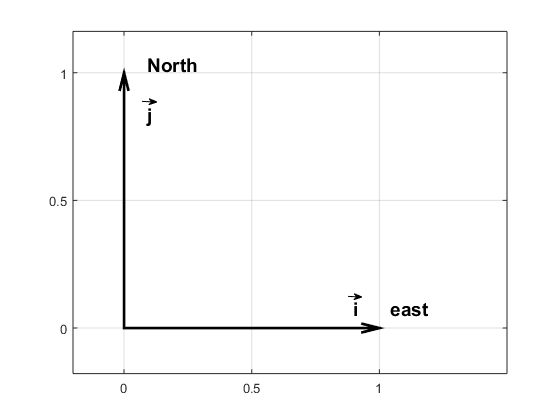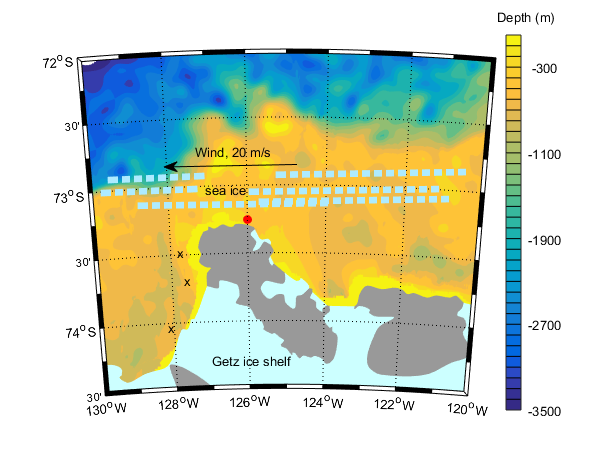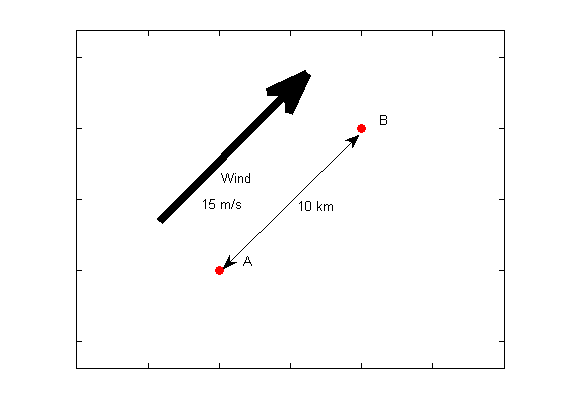# Exercises: Wind and water

Wind and currents have a strength and a direction and therefor use vectors to represent them, for example: $$\vec{u}=u\vec{i} + v\vec{j}$$. $$\vec{i}$$ and $$\vec{j}$$ are unit vecors pointing towards east and north (see figure) while $$u$$ and $$v$$ gives the length of the vectors or the strength of the current in that direction If the wind blows towards the north with 10 m/s we have $$\vec{u}=10\vec{i}$$; if it blows towards south $$\vec{u}=-10\vec{i}$$; and if it blows towards the northeast we get $$\vec{u}=7.1\vec{i}+7.1\vec{j}$$.Unit vecor i has length 1 and point to the east. The unit vector j has length 1 and points to the north.

The ice conditions change constantly due to wind and currents – so the captain must follow the evolution through e.g. satellite images so that we don’t get “caught” by the drifting ice. (Araon is an ice breaker, but breaking ice is slow and very fuel consuming) . The sea ice is realtively thin and its motion is most affected by the wind – a rule of thumb is that the ice more with about 2% of the speed of the wind and about 30 degrees to the left of the wind (in the north it moves to the right of the wind).

## Exercise 1

The wind is blowing 20 m/s towards the west and the drifting ice is 5 km from land.

a)Express the motion of the wind and the ice on vector format

b) How far southward will the ice move in an hour?

c) How fast does Araon have to drive to make past the peninsula to the moorings on the other side before the ice closes the passage? Will we make it?Map over the Amundsen Sea. Light blue areas are covered by ice shelves. grey areas are land. Araon (the red dot) want to get over to the western front of the Getz ice shelf to deploy moorings (crosses), but the ice is moving towardsland… will we get past the peninsula in time, or will we get stuck in the ice?

## Exercise 2

The wind exerts a stress $$(\vec{\tau}=\tau_x\vec{i} + \tau_y\vec{j})$$ on the sea surface that sets up a current in the water.The size of the stress can be calculated if we know the strength of the wind: $$\vec{\tau}=C_{D}\left|\vec{u}\right|\vec{u}$$, where $$\vec{u}=u\vec{i}+v\vec{j}$$ is the wind at 10 m height.

1. a) If it is blowing 10 m/s towards the north and $$C_D=1.6\times10^{-3}$$, how large is the stress then? In which direction is it acting?
2. b) We know that the size of $$C_D$$ change when there is ice on the water and scientist have found that $$C_D=10^{-3}\times(1.5+2.233C_i-2.333C_i^2)$$ where $$C_i$$ is the fraction of the sea surface that is covered by ice (the ice concentration). For what ice concentration is $$C_D$$ largest? smallest? How large was the ice concentration in the exercise above?

## Exercise 3

During their expedition on FRAM (1893-1896) the Norwegian oceanographer Nansen observed that the ice was drifting to the right of the wind (he was in the Arctic) and he asked his friend Vilhelm Bjerknes to get one of his students to study the problem. It was the Swedish scientist Vagn Valfrid Ekman who ended up explaining that the motion of ice is an effect of friction and the rotation of the Earth. Ekman developed a theory describing how the current that is set up by the wind behaves. The current changes with depth and we can write: $$\vec{u(z)}=u(z)\vec{i} + v(z)\vec{j}$$.

The size of $$u(z)$$ and $$v(z)$$ can be calculated from the following formaulas (which are not as complicated as they look!)

$$u(z)=\frac{\sqrt{2}}{1000fd} e^{z/d}\left[\tau_x cos(z/d-\pi/4) -\tau_y sin(z/d-\pi/4)\right]$$

$$v(z)=\frac{\sqrt{2}}{1000fd} e^{z/d}\left[\tau_x sin(z/d-\pi/4) +\tau_y sin(z/d-\pi/4)\right]$$

where $$z$$ is height (so the depths are negative), $$f=1.46\times10^{-4}sin(latitud)$$ is the Coriolis faktorn (caused by the rotation of the Earth) and $$d=\sqrt{\frac{2\nu}{\left|f\right|}}$$ is the thickness of the layer that is affected by the wind. We often call this layer the “Ekman layer”. $$\nu=10^{-2}m^2/s$$ is the viscosity* – the “thickness” of the water (syrup has a higher viscosity than water**). The theory is well known amongst oceanographers and is referred to as the Ekman spiral.

* The molecular viscosity of water is much lower $$\nu=10^{-6}m^2/s$$, but turbulence and eddies make the effective viscosity in the ocean much higher

** see e.g. https://no.wikipedia.org/wiki/Viskositet for an animation showing the effect of viscosity

## Exercise 4

1. What is the value of the Coriolis factor in the Amundsen Sea? In Bergen?
2. How thick is the Ekman layer? An iceberg can be several hundred of meters thick – do you think it is affected by the current set up by the wind?
3. If the wind is blowing towards west with 15 m/s and there is no ice – how large is then $$\tau_x$$ and $$\tau_y$$? (See exercise 2)
4. In what direction (and with what speed) is the water in the surface (z=0) flowing? At the Ekman depth(z=-d)? At what depth is the current flowing in the opposite direction to the wind?
5. Try to plot the current! Why do you think we talk about an Ekman spiral?
6. When we send down our LADCP*** we get current profiles with a resolution of 8m (i.e we get one measurements for the current every 8 m) Do you think we are able to observe the Ekman spiral? Why/why not?

*** the LADCP is an instrument that we send down together with the CTD all the way to the bottom and that measures the current in the water on its way down (and up) so that we get profiles of current in addition to profiles of temperature and salinity.Illustration to exercise 4. The wind is blowing parallel to the line A-B. In what direction is the Ekman transport? what would have happened if A-B was the coast line?

## Exercise 5

If you sum up (integrate) the current set up by the wind in the Ekman layer we get the transport, that is how much water that is moved in what direction due to the wind. We often call that the Ekman transport $$\vec{Q_{Ekman}}=U\vec{i}+V\vec{j}$$, where

$$U=\frac{10^{-3}}{f}\tau_y$$

$$V=-\frac{10^{-3}}{f}\tau_x$$

The unit of U and V is $$m^3/m/s$$ (or $$m^2/s$$) and they give the amount of water that is flowing by per meter per second. The expression for $$\tau$$ is given in exercise 2.

1. If it is blowing 15 m/s to the northeast and there is no ice, how larger is then U and V? In what direction is the water transported?
2. How large is the angle between the wind and the transport?
3. We have two buoys (see figure below) that are place 10 km apart and where a line between the buoys are parallel to the wind. How much water flows between the buoys per second? What do you think would have happened I the line between A and B would have been the coast?
4. In what direction do you think the water is blowing when the Bryggen in Bergen is flooded (the tides are also important, off cpurse!)
5. Along the coast of the Antarctic continent the wind is normally blowing towards the west – further north (60S) the wind is blowing to the east. Make a sketch showing the direction of the wind and the Ekman currents. What do you think happens in the middle? Discuss with your friends before reading the answer at the bottom of the page.

## Convergence and divergence in the ocean

When the wind and thus the Ekman transport changes direction (or magnitude) from one region to another we get “convergence” or divergence. When we have divergence the surface water in an area “disappears” (i.e. it moves away and is not replenished) and new surface water must be provided from below. The water from depth is sucked up to the surface by the wind. In areas where this happens continuously (e.g. around Antarctica) we find large biological activity, since the water from depth is rich in nutrients. If you want to read more, then google e.g. “Wind driven upwelling”.